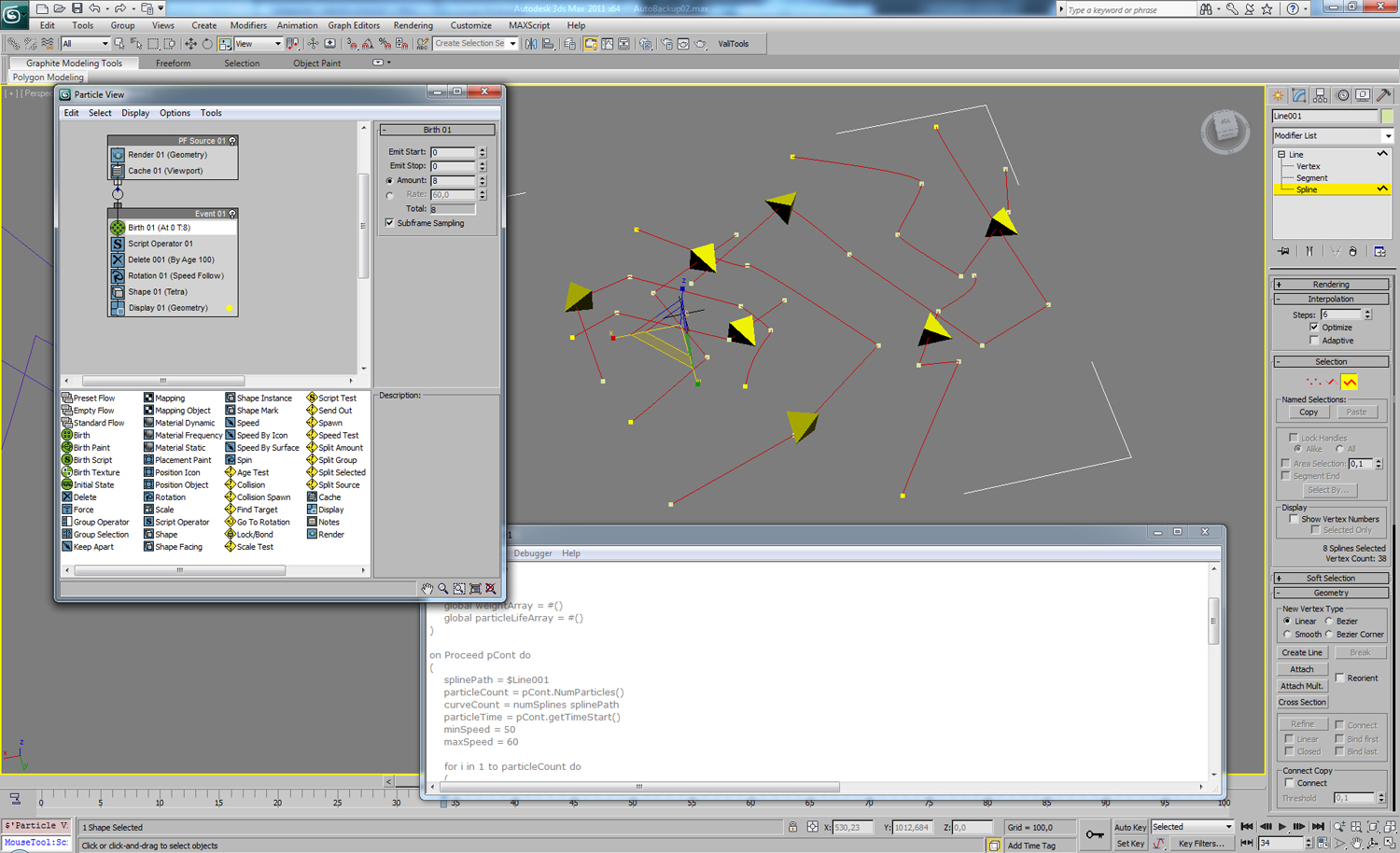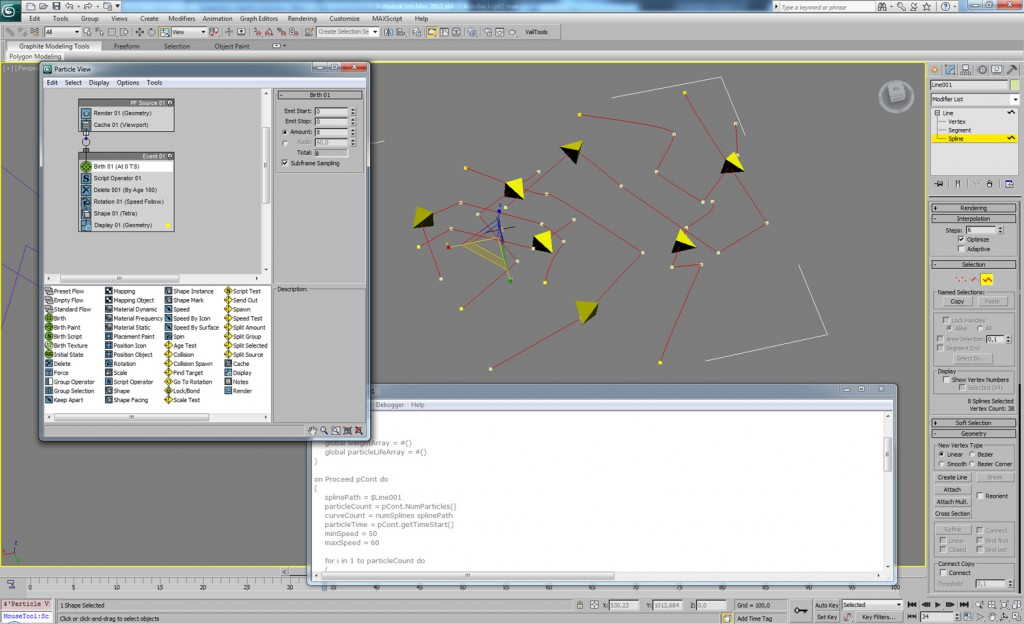### valentin schwind

human-computer interaction## PFlow Script Operator: Particles follow Splines

Unfortunately there is no Spline-Operator for Particle Flow in 3ds Max and Path-Follow SpaceWarp don’t work with the Force-Operator in PFlow. So we need a particle flow script to control the position of each particle with splines. Here is my solution of this problem.

1. Create a group of shapes (e.g. 20 splines) and attach them to one object. Copy the object name.
2. Create a Standard-PFlow-System.
3. Set the “Amount” of particles in the Birth-Operator equal to the amount of spline curves in the shape object (in this case: 20) and turn down “Emit Start” and “Emit Stop” to 0.
4. Delete the Position Icon and Speed-Operator of the first event
5. Add a “Delete” operator and set your life span to a particle age (e.g. 100)
6. Add a Script-Operator with following script and paste the name of your target spline in line 18:
```on ChannelsUsed pCont do
(
pCont.useAge = true
pCont.useLifespan = true
pCont.useSpeed = true
pCont.usePosition = true
)

on Init pCont do
(
seed 12345
global weightArray = #()
global particleLifeArray = #()
)

on Proceed pCont do
(
splinePath = \$Line001
particleCount = pCont.NumParticles()
curveCount = numSplines splinePath
particleTime = pCont.getTimeStart()
minSpeed = 50
maxSpeed = 60

for i in 1 to particleCount do
(
pCont.particleIndex = i

if weightArray[pCont.particleID] == undefined do
(
weight = for s = 1 to curveCount collect 1.0
sum = 0
for s in weight do sum += s
weightArray[pCont.particleID] = for s in weight collect s/sum
)

if particleLifeArray[pCont.particleID] == undefined do
(
for s = 1 to curveCount do
(
pathLength = (curveLength splinePath s) / random minSpeed maxSpeed
particleLifeArray[pCont.particleID] = pathLength
)
)

actualSplineProgress = mod  (pCont.particleAge.frame / particleLifeArray[pCont.particleID]) 1.0
nextSplineProgress = mod ((pCont.particleAge.frame + 1) / particleLifeArray[pCont.particleID]) 1.0

actualPosition = [0,0,0]
nextPosition = [0,0,0]

for s = 1 to curveCount do
(
at time particleTime
(
actualPosition += (lengthInterp splinePath i actualSplineProgress) * weightArray[pCont.particleID][s]
nextPosition += (lengthInterp splinePath i nextSplineProgress) * weightArray[pCont.particleID][s]
)
)
pCont.particleSpeed = (nextPosition-actualPosition) / TicksPerFrame
pCont.particlePosition = nextPosition
)
)

on Release pCont do
(

)```

Press CTRL-E to evaluate your script. You can open the MAXScript Listener Window to see all values. You can use the “Cache” operator in your PF Source for faster results.Cheers!

Vali